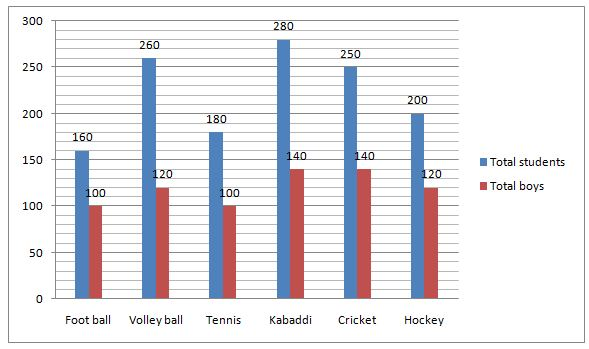# NIACL AO Prelims – Quantitative Aptitude Questions Day- 74

Dear Readers, Bank Exam Race for the Year 2019 is already started, To enrich your preparation here we have providing new series of Practice Questions on Quantitative Aptitude – Section. Candidates those who are preparing for NIACL AO Prelims 2019 Exams can practice these questions daily and make your preparation effective.

[WpProQuiz 4846]

Directions (1 – 5): Study the following information carefully and answer the given questions:

The following bar graph shows the total number of students (Boys + Girls) and the number of boys participated in the different games.1) Total number of girls participated in Football is what percentage of total number of boys participated in Tennis?

a) 75 %

b) 60 %

c) 50 %

d) 40 %

e) None of these

2) Find the ratio between the total number of students participated in Volleyball and Tennis together to that of total number of girls participated in Kabaddi and Cricket together?

a) 35: 19

b) 27: 11

c) 15: 7

d) 44: 25

e) None of these

3) Find the difference between the total number of girls to that of total number of boys participated in Foot ball and Hockey together?

a) 80

b) 60

c) 50

d) 70

e) None of these

4) Find the average number of girls participated in Volley ball, Tennis and Cricket together?

a) 90

b) 120

c) 110

d) 70

e) None of these

5) Total number of students participated in Football is approximately what percentage more/less than the total number of boys participated in Kabaddi?

a) 30 % less

b) 15 % less

c) 45 % more

d) 15 % more

e) 30 % more

6) There are two Jars containing the mixture of milk and water in the ratio of 4:3 and 3:2 respectively. If the mixture of two Jars is mixed, then the quantity of water in the third Jar is 58 litres. Find the quantity of milk in the third Jar?

a) 80 litres

b) 82 litres

c) 78 litres

d) 84 litres

e) None of these

7) The ratio of the radius of a circle and the side of a square is 2:1. Perimeter of a rectangle is 68cm and the length of the rectangle is equal to the side of the square. The length of the rectangle is 2 cm more than the breadth of the rectangle. Find the area of the circle?

a) 1296∏

b) 1266∏

c) 1286∏

d) 1276∏

e) None of these

8) The average score of team A and team B is 220, the average score of team A and team C is 320 and the average score of team C and team B is 180. Find the average score of team C

a) 280

b) 260

c) 250

d) 220

e) None of these

9) A and B enter into partnership in the investment ratio of 2:1. At the end of 8 months, A left the business. If they receive the profit ratio of 4:5, then find how long is B’s investment used?

a) 18 months

b) 20 months

c) 16 months

d) 15 months

e) None of these

10) Train A crosses a pole in 18 seconds and another train B crosses a pole in 54 seconds. The length of train A is 50% of the length train B. What is the ratio of the speed of train A to train B?

a) 3:2

b) 2:5

c) 4:5

d) 5:6

e) None of these

Directions (1-5):

Total number of girls participated in Football = 160 – 100 = 60

Total number of boys participated in Tennis = 100

Required % = (60/100)*100 = 60 %

The total number of students participated in Volleyball and Tennis together

= > 260 + 180 = 440

The total number of girls participated in Kabaddi and Cricket together

= > (280 – 140) + (250 – 140)

= > 140 + 110 = 250

Required ratio = 440: 250 = 44: 25

The total number of girls participated in Foot ball and Hockey together

= > (160 – 100) + (200 – 120)

= > 60 + 80 = 140

The total number of boys participated in Foot ball and Hockey together

= > 100 + 120 = 220

Required difference = 220 – 140 = 80

The total number of girls participated in Volley ball, Tennis and Cricket together

= > (260 – 120) + (180 – 100) + (250 – 140)

= > 140 + 80 + 110 = 330

Required average = 330/3 = 110

Total number of students participated in Football = 160

Total number of boys participated in Kabaddi = 140

Required % = [(160 – 140)/140]*100 = 15 % more

Milk and water ratio in the third Jar= (4/7+3/5): (3/7+2/5)

=41:29

Milk=41/29*58=82 litres

(l – b) =2 ——— (1)

2(l + b) =68

(l + b) =34 ——– (2)

(1)+(2)

2l=36

= > l =18 cm

Side of the square=18 cm

Area of the circle=∏*36*36=1296∏

A+B=440 ———– (1)

A+C=640 ————- (2)

C+B=360 ——– (3)

B=360-C

A+360-C=440

A-C=80 — (4)

(2)+(4)

2A=720

A=360

C=360 – 80=280

(2*8)/(1*x)=4/5

16/x=4/5

X=20 months

Speed of train A be S1 km/hr and speed of train B be S2 km/hr

Train B length=x

X*50/100=S1*5/18*18

(x/2)=5S1

S1=x/10

x=S2*5/18*54

x=15S2

S2=x/15

Ratio=x/10:x/15=15:10=3:2